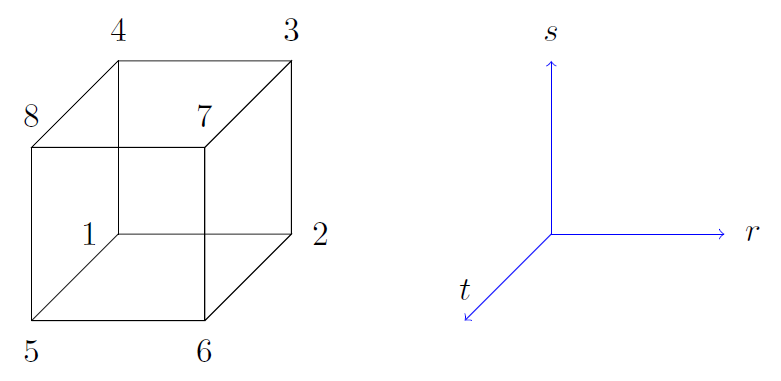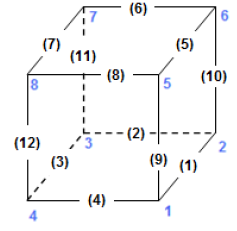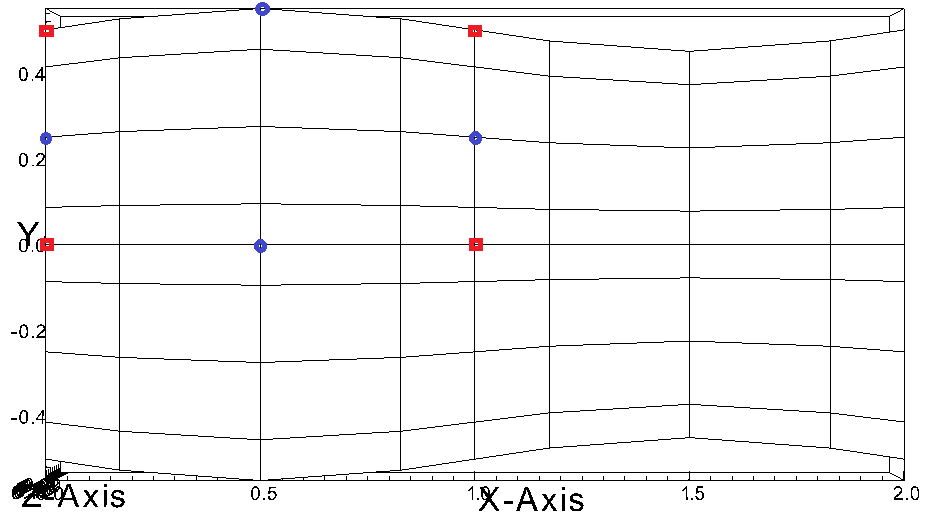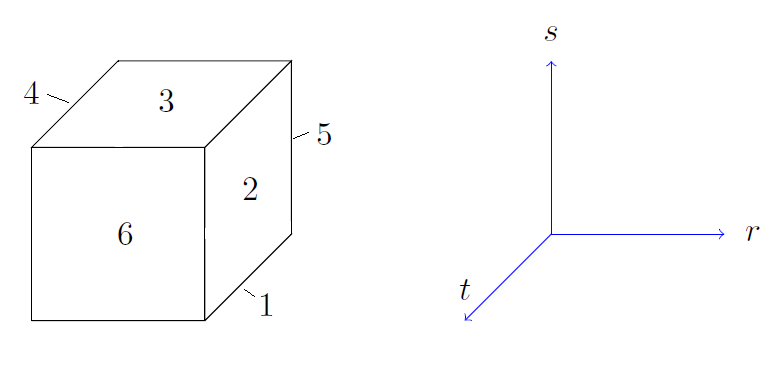# Case Files¶

Each simulation is defined by six required case files:

• SESSION.NAME
• par (runtime parameters)
• re2 (mesh and boundaries)
• usr (user defined functions for inital/boundary condition etc.)
• SIZE (parameters for static memory allocation)
• map/ma2 (element processes mapping)

Additional optional case files may be generated or included:

• f%05d (solution data)
• his (probing point data)

## SESSION.NAME¶

To run Nek5000, each simulation must have a SESSION.NAME file. This file is read in by the code and gives the path to the relevant files describing the structure and parameters of the simulation. The SESSION.NAME file is a file that contains the name of the simulation and the full path to supporting files. For example, to run the eddy example from the repository, the SESSION.NAME file would look like:

eddy_uv
/home/user_name/Nek5000/short_tests/eddy/


Note that this file is generated automatically by the nek, nekb, nekmpi and nekbmpi scripts at runtime.

## Parameter File (.par)¶

The simulation paramaters are defined in the .par file. The keys are grouped in different sections and a specific value is assigned to each key. The .par file follows the structure exemplified below.

#
# nek parameter file
#

[SECTION]
key = value
...

[SECTION]
key = value
...


The sections are:

• GENERAL (mandatory)
• PROBLEMTYPE
• MESH
• VELOCITY
• PRESSURE (required for velocity)
• TEMPERATURE
• SCALAR%%
• CVODE

When scalars are used, the keys of each scalar are defined under the section SCALAR%% varying between SCALAR01 and SCALAR99. The descripton of the keys of each section is given in the following tables (all keys/values are case insensitive). The value assigned to each key can be a user input (e.g. a <real> value) or one of the avaliable options listed in the tables below. Values in parentheses denote the default value.

Table 7 GENERAL keys in the .par file
Key
Value(s)
Description
startFrom
<string>
Absolute/relative path of the field file
to restart the simulation from
stopAt
(numSteps)
endTime
Stop mode
endTime
<real>
Final physical time at which we want to
our simulation to stop
numSteps
<real>
Number of time steps instead of specifying
final physical time
dt
<real>
Specifies the step size or in case of a
a variable time step the maximum step size
variableDT
(no)
yes
Controls if the step size will be adjusted
to match the targetCFL
targetCFL
<real>
Sets stability/target CFL number for
OIFS or variable time steps
(fixed to 0.5 for standard extrapolation
writeControl
(timeStep)
runTime
Specifies whether checkpointing is based
on number of time steps or physical time
writeInterval
<real>
Checkpoint frequency in time steps or
physical time
filtering
(none)
explicit
hpfrt
Specifies the filtering method
filterModes
<int>
Specifies the number of modes filtered as
an alternative to specifying the cutoff
ratio
Note: requires the use of at least 2 modes
filterCutoffRatio
<real>
Ratio of modes not affected by the filter
Use i.e. for stabilization or LES 0.9/0.65
as an alternative to specifying the number
of modes explicitly
filterWeight
<real>
Sets the filter strength of transfer
function of the last mode (explicit) or the
relaxation parameter in case of hpfrt
writeDoublePrecision
no
(yes)
Sets the precision of the field files
writeNFiles
(1)
Sets the number of output files
By default a parallel shared file is used
dealiasing
no
(yes)
Enable/diasble over-integration
timeStepper
BDF1
(BDF2)
BDF3
Time integration order
extrapolation
(standard)
OIFS
Extrapolation method
optLevel
(2)
Optimization level
logLevel
(2)
Verbosity level
userParam%%
<real>
User parameter (can be accessed through
uparam(%) array in .usr
Table 8 PROBLEMTYPE keys in the .par file
Key
Value(s)
Description
equation
(incompNS)
lowMachNS
steadyStokes
incompLinNS
incompLinAdjNS
incompMHD
compNS
Specifies equation type
axiSymmetry
(no)
yes
Axisymmetric problem
swirl
(no)
yes
Enable axisymmetric azimuthal velocity
component (stored in temperature field
cyclicBoundaries
(no)
yes
Sets cyclic periodic boundaries
numberOfPerturbations
(1)
Number of perturbations for linearized NS
solveBaseFlow
(no)
yes
Solve for base flow in case of linearized NS
variableProperties
(no)
yes
Enable variable transport properties
stressFormulation
(no)
yes
Enable stress formulation
dp0dt
(no)
yes
Enable time-varying thermodynamic pressure
Table 9 COMMON keys for all field variables in the .par file
Key
Value(s)
Description
residualTol
<real>
Residual tolerance used by solver (not for CVODE)
residualProj
(no)
yes
Controls the residual projection
writeToFieldFile
no
(yes)
Controls if fields will be written on output
Table 10 MESH keys in the .par file
Key
Value(s)
Description
motion
(none)
user
elasticity
Mesh motion solver
viscosity
(0.4)
Diffusivity for elasticity solver
numberOfBCFields
(nfields)
Number of field variables which have a boundary
condition in .re2 file
firstBCFieldIndex
(1 or 2)
Field index of the first BC specified in .re2
file
Table 11 VELOCITY keys in the .par file
Key
Value(s)
Description
viscosity
<real>
Dynamic viscosity
A negative value sets the Reynolds number
density
<real>
Density
Table 12 PRESSURE keys in the .par file
Key
Value(s)
Description
preconditioner
(semg_xxt)
semg_amg
Preconditioning method
First time usage of AMG will write three
dump files to disc. Subsequently please run
the amg_hypre tool to create the setup files
required for the AMG solver initialization
Table 13 COMMON keys for temperature and scalar fields in the .par file
Key
Value(s)
Description
solver
(helm)
cvode
none
Solver for scalar
advection
no
(yes)
absoluteTol
<real>
Absolute tolerance used by CVODE
Table 14 TEMPERATURE keys in the .par file
Key
Value(s)
Description
ConjugateHeatTransfer
(no)
yes
Controls conjugate heat transfer
conductivity
<real>
Thermal conductivity
rhoCp
<real>
Product of density and heat capacity

Note: [TEMPERATURE] solver = none is incompatible with [PROBLEMTYPE] equation = lowMachNS without defining a custom thermal divergence in the usr file.

Table 15 SCALAR%% keys in the .par file
Key
Value(s)
Description
density
<real>
Density
diffusivity
<real>
Diffusivity
Table 16 CVODE keys in the .par file
Key
Value(s)
Description
relativeTol
<real>
Relative tolerance (applies to all scalars)
stiff
no
(yes)
Controls if BDF or Adams Moulton is used
preconditioner
(none)
user
Preconditioner method
dtMax
<real>
Maximum internal step size
Controls splitting error of velocity
scalar coupling (e.g. set to 1-4 dt)

## Mesh File (.re2)¶

Stores the mesh and boundary condition.

TODO: Update to re2

### Element data¶

Table 17 Geometry description in .rea file
ELEMENT 1 [ 1A] GROUP 0
Face {1,2,3,4}
$$x_{1,\ldots,4}=$$ 0.000000E+00 0.171820E+00 0.146403E+00 0.000000E+00
$$y_{1,\ldots,4}=$$ 0.190000E+00 0.168202E+00 0.343640E+00 0.380000E+00
$$z_{1,\ldots,4}=$$ 0.000000E+00 0.000000E+00 0.000000E+00 0.000000E+00
Face {5,6,7,8}
$$x_{5,\ldots,8}=$$ 0.000000E+00 0.171820E+00 0.146403E+00 0.000000E+00
$$y_{5,\ldots,8}=$$ 0.190000E+00 0.168202E+00 0.343640E+00 0.380000E+00
$$z_{5,\ldots,8}=$$ 0.250000E+00 0.250000E+00 0.250000E+00 0.250000E+00

Following the header, all elements are listed. The fluid elements are listed first, followed by all solid elements if present.

The data following the header is formatted as shown in Table 17. This provides all the coordinates of an element for top and bottom faces. The numbering of the vertices is shown in Fig. Fig. 13. The header for each element as in Table 17, i.e. [1A] GROUP is reminiscent of older Nek5000 format and does not impact the mesh generation at this stage.Fig. 13 Geometry description in .rea file (sketch of one element ordering - Preprocessor corner notation)

### Curved Sides¶

This section describes the curvature of the elements. It is expressed as deformation of the linear elements. Therefore, if no elements are curved (if only linear elements are present) the section remains empty.

The section header may look like this:

640 Curved sides follow IEDGE,IEL,CURVE(I),I=1,5, CCURVE


Curvature information is provided by edge and element. Therefore up to 12 curvature entries can be present for each element. Only non-trivial curvature data needs to be provided, i.e., edges that correspond to linear elements, since they have no curvature, will have no entry. The formatting for the curvature data is provided in Table 18.

Table 18 Curvature information specification
IEDGE IEL CURVE(1) CURVE(2) CURVE(3) CURVE(4) CURVE(5) CCURVE
9 2 0.125713 -0.992067 0.00000 0.00000 0.00000 m
10 38 0.125713 -0.992067 3.00000 0.00000 0.00000 m
1 40 1.00000 0.000000 0.00000 0.00000 0.00000 C

There are several types of possible curvature information represented by CCURVE. This include:

• ‘C’ stands for circle and is given by the radius of the circle, in CURVE(1), all other compoentns of the CURVE array are not used but need to be present.
• ‘s’ stands for sphere and is given by the radius and the center of the sphere, thus filling the first 4 components of the CURVE array. The fifth component needs to be present but is not utilized.
• ‘m’ is given by the coordinates of the midside-node, thus using the first 3 components of the CURVE array, and leads to a second order reconstruction of the face. The fourth and fifth components need to be present but are not utilized.

Both ‘C’ and ‘s’ types allow for a surface of as high order as the polynomial used in the spectral method, since they have an underlying analytical description, any circle arc can be fully determined by the radius and end points. However for the ‘m’ curved element descriptor the surface can be reconstructed only up to second order. This can be later updated to match the high-order polynomial after the GLL points have been distributed across the boundaries. This is the only general mean to describe curvature currrently in Nek5000 and corresponds to a HEX20 representation.Fig. 14 Edge numbering in .rea file, the edge number is in between parenthesis. The other numbers represent vertices.Fig. 15 Example mesh - with curvature. Circular dots represent example midsize points.

### Boundaries¶

Boundaries are specified for each field in sequence: velocity, temperature and passive scalars. The section header for each field will be as follows (example for the velocity):

***** FLUID   BOUNDARY CONDITIONS *****


and the data is stored as illustarted in Table 19. For each field boundary conditions are listed for each face of each element.

Boundary conditions are given in order per each element, see Table 19 column IEL, and faces listed in ascending order 1-6 in column IFACE. Note that the header in Table 19 does not appear in the actual .rea.

The ordering for faces each element is shown in Fig. 16. A total equivalent to $$6N_{field}$$ boundary conditions are listed for each field, where $$N_{field}$$ is the number of elements for the specific field. $$N_{field}$$ is equal to the total number of fluid elements for the velocity and equal to the total number of elements (including solid elements) for temperature. For the passive scalars it will depend on the specific choice, but typically scalars are solved on the temeprature mesh (solid+fluid).Fig. 16 Face ordering for each element.

Each BC letter condition is formed by three characters. Common BCs include:

• E - internal boundary condition. No additional information needs to be provided.
• SYM - symmetry boundary condition. No additional information needs to be provided.
• P - periodic boundary conditions, which indicates that an element face is connected to another element to establish a periodic BC. The connecting element and face need be to specified in CONN-IEL and CONN-IFACE.
• v - imposed velocity boundary conditions (inlet). The value is specified in the user subroutines. No additional information needs to be provided in the .rea file.
• W - wall boundary condition (no-slip) for the velocity. No additional information needs to be provided.
• O - outlet boundary condition (velocity). No additional information needs to be provided.
• t - imposed temperature boundary conditions (inlet). The value is specified in the user subroutines. No additional information needs to be provided in the .rea file.
• f - imposed heat flux boundary conditions (temperature). The value is specified in the user subroutines. No additional information needs to be provided in the .rea file.
• I - adiabatic boundary conditions (temeperature). No additional information needs to be provided.

Many of the BCs support either a constant specification or a user defined specification which may be an arbitrary function. For example, a constant Dirichlet BC for velocity is specified by V, while a user defined BC is specified by v. This upper/lower-case distinction is used for all cases. There are about 70 different types of boundary conditions in all, including free-surface, moving boundary, heat flux, convective cooling, etc. The above cases are just the most used types.

Table 19 Formatting of boundary conditions input.
CBC IEL IFACE CONN-IEL CONN-IFACE
E 1 1 4.00000 3.00000 0.00000 0.00000 0.00000
.. .. .. .. .. .. .. ..
W 5 3 0.00000 0.00000 0.00000 0.00000 0.00000
.. .. .. .. .. .. .. ..
P 23 5 149.000 6.00000 0.00000 0.00000 0.00000

## User Routines File (.usr)¶

This file implements the the user interface to Nek5000. What follows is a brief description of the available subroutines.

### uservp¶

This function can be used to specify customized or solution dependent material properties.

Example:

if (ifield.eq.1) then
udiff  = a * exp(-b*temp) ! dynamic viscosity
utrans = 1.0              ! density
else if (ifield.eq.2) then
udiff  = 1.0              ! conductivity
utrans = 1.0              ! rho*cp
endif


### userf¶

This functions sets the source term (which will be subsequently be multiplied by the density) for the momentum equation.

Example:

parameter(g = 9.81)

ffx = 0.0
ffy = 0.0
ffz = -g ! gravitational acceleration


### userq¶

This functions sets the source term for the energy (temperature) and passive scalar equations.

### userbc¶

This functions sets boundary conditions. Note, this function is only called for special boundary condition types and only for points on the boundary surface.

### useric¶

This functions sets the initial conditions.

### userchk¶

This is a general purpose function that gets executed before the time stepper and after every time step.

### userqtl¶

This function can be used to specify a cutomzized thermal diveregence for the low Mach solver. step.

### usrdat¶

This function can be used to modify the element vertices and is called before the spectral element mesh (GLL points) has been laid out.

### usrdat2¶

This function can be used to modify the spectral element mesh. The geometry information (mass matrix, surface normals, etc.) will be rebuilt after this routine is called.

### usrdat3¶

This function can be used to initialize case/user specific data.

## SIZE¶

SIZE file defines the problem size, i.e. spatial points at which the solution is to be evaluated within each element, number of elements per processor etc. The SIZE file governs the memory allocation for most of the arrays in Nek5000, with the exception of those required by the C utilities. The basic parameters of interest in SIZE are:

• ldim = 2 or 3. This must be set to 2 for two-dimensional or axisymmetric simulations (the latter only partially supported) or to 3 for three-dimensional simulations.
• lx1 controls the polynomial order of the solution, $$N = {\tt lx1-1}$$.
• lxd controls the polynomial order of the (over-)integration/dealiasing. Strictly speaking $${\tt lxd=3 * lx1/2}$$ is required but often smaller values are good enough.
• lx2 = lx1 or lx1-2 and is an approximation order for pressure that determines the formulation for the Navier-Stokes solver (i.e., the choice between the $$\mathbb{P}_N - \mathbb{P}_N$$ or $$\mathbb{P}_N - \mathbb{P}_{N-2}$$ spectral-element methods).
• lelg, an upper bound on the total number of elements in your mesh.
• lpmax, a maximum number of processors that can be used
• lpmin, a minimum number of processors that can be used (see also Memory Requirements).
• ldimt, an upper bound on a number of auxilary fields to solve (temperature + other scalars, minimum is 1).

The optional upper bounds on parameters in SIZE are (minimum being 1 unless otherwise noted):

• lhis, a maximum history (i.e. monitoring) points.
• maxobj, a maximum number of objects.
• lpert, a maximum perturbations.
• toteq, a maximum number of conserved scalars in CMT (minimum could be 0).
• nsessmax, a maximum number of (ensemble-average) sessions.
• lxo, a maximum number of points per element for field file output ($${\tt lxo \geq lx1}$$).
• lelx, lely, lelz, a maximum number of element in each direction for global tensor product solver and/or dimentions.
• mxprev, a maximum dimension of projection space (e.g. 20).
• lgmres, a maximum dimension of Krylov space (e.g. 30).
• lorder, a maximum order of temporal discretization (minimum is2 see also characteristic/OIFS method).
• lelt determines the maximum number of elements per processor (should be not smaller than nelgt/lpmin, e.g. lelg/lpmin+1).
• lx1m, a polynomial order for mesh solver that should be equal to lx1 in case of ALE and in case of stress-formulation (=1 otherwise).
• lbelt determines the maximum number of elements per processor for MHD solver that should be equalt to lelt (=1 otherwise).
• lpelt determines the maximum number of elements per processor for linear stability solver that should be equalt to lelt (=1 otherwise).
• lcvelt determines the maximum number of elements per processor for CVODE solver that should be equalt to lelt (=1 otherwise).
• lfdm equals to 1 for global tensor product solver (that uses fast diagonalization method) being 0 otherwise.

Note that one also need to include the following line to SIZE file:

include 'SIZE.inc'


## Restart/Output files (.f#####)¶

Field files are used to read/write physical fields in both serial and parallel. They have extension .f##### where # is a numeral. The file format is unique to Nek5000 and is described in this section.

The file is composed of:

• Global element IDs, coordinates, and field data

The header provides information about the types, sizes, and layout of the field data. The header is a fixed size of 132 bytes. Its data elements are encoded as either ASCII or binary values. In the table below, the offsets and widths are measured in bytes. Note that consecutive entries are separated by a single byte, which is the ASCII space character. Finally, note that the data entries do not require all 132 bytes.

Some elements that require additional explanation are:

• nz: This is the number of GLL gridpoints in the z-direction. If equal to 1, the field data were produced for a 2D simulation. If > 1, the data were produced for a 3D simulation.
• rdcode: Specifies the type and ordering of fields that are present in this file. The code can consist of the following. All fields are optional, but if present, they are expected to appear in this order.
• X : Coordinates
• U : Velocity
• P : Pressure
• T : Temperature
• S#: Passive scalar(s). # is a numeral that specifies the number of different passive scalars.
• test value: When Nek5000 writes the header to file, it writes the specific value 6.54321 as a test value. When the file is later read – possibly on a different computer – the test value is read and compared to the expected value. If the values match, then the computer that wrote the file and the computer that is now reading the file use the same endianness for floating-point numbers. If not, the computers have different endianness. In that case, the floating-point data should be byte-swapped by the computer reading the data.
Name Offset Width Encoding Datatype Description
tag 0 4 ASCII text The string #std. Tags the start of the fil
wdsize 5 1 ASCII integer Floating-point precision of field data (in bytes)
nx 7 2 ASCII integer Number of GLL points per element in x direction
ny 10 2 ASCII integer Number of GLL points per element in y direction
nz 13 2 ASCII integer Number of GLL points per element in z direction
nelt 16 10 ASCII integer Number of elements in this file
nelgt 27 10 ASCII integer Number of global elements
time 38 20 ASCII decimal Absolute simulation time of this file’s state
iostep 59 9 ASCII integer I/O timestep of this file’s state
fid 69 6 ASCII integer Index of this file (when using multi-file output)
nfileoo 76 6 ASCII integer Number of files produced at this I/O step
rdcode 83 10 ASCII text Specifies which fields contained in this file
p0th 94 15 ASCII decimal Thermodynamic pressure
if_press_mesh 110 1 ASCII text States whether pressure mesh is being used
test_value 112 4 binary 32-bit float The decimal 6.54321. Used to test endianness.

The global element IDs, coordinates, and field data start at offset 136 bytes. Integer data are always 32-bit. The precision of floating-point data is inferred from the value of wdsize (see above). The number of dimensions (ndims) is inferred from nz (see above). The global element IDs are required, but the coordinates and any field data are optional. Their presence of coordinates and field data are inferred from rdcode, as described above.

Value Datatype Shape
Global element IDs integer (nelt)
Coordinates float (nelt, ndims, nx * ny * nz)
Velocity float (nelt, ndims, nx * ny * nz)
Pressure float (nelt, nx * ny * nz)
Temperature float (nelt, nx * ny * nz)
Passive scalars float (nscalars, nelt, nx * ny * nz)